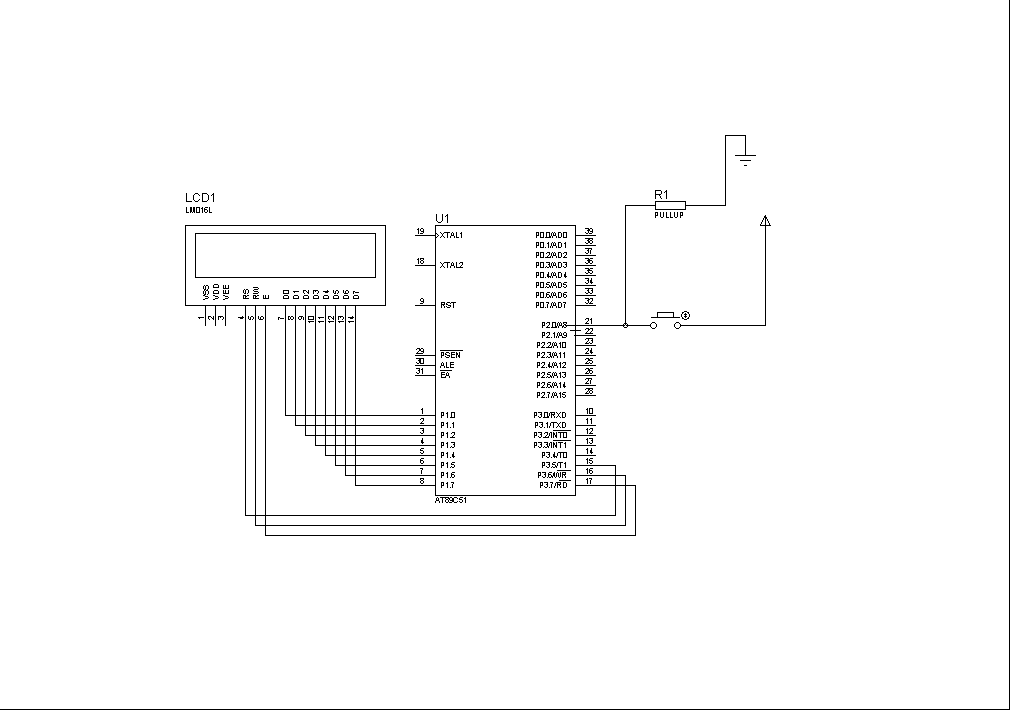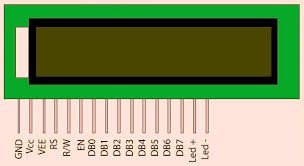﻿ HOW TO INTERFACE LCD WITH 8051 MICROCONTROLLERBack

## HOW TO INTERFACE LCD WITH 8051 MICROCONTROLLERThe LCD standardrequires 3 control lines and 8 I/O lines for the data bus.

8 datapins D7:D0

Bi-directionaldata/command pins.
Alphanumeric characters are sent in ASCII format.

RS: Register Select

RS= 0 -> Command Register is selected
RS = 1 -> Data Register is selected

0 -> Write,  1 -> Read

E:Enable (Latch data)

Usedto latch the data present on the data pins.
A high-to-low edge is needed to latch the data.

The 8 data lines are connected to PORT 1 of 8051microcontroller. The three control lines( RS,RW and EN ) are connected to PORT3.5,3.6 and 3.7 respectively.

LCD SUB PROGRAM:

sbit RS=P3^5;
sbit rw=P3^6;
sbit EN=P3^7;

void string(const char *q);
void delay(unsigned char a);
void lcd_data(unsigned char a);
void lcd_cmd(unsigned char b);
void delay(unsigned char a);
void lcd_init();

void string(const char *q)
{
while(*q)
{
lcd_data(*q++);
}
}
//void delay();

void lcd_data(unsigned char a)
{
RS=1;
rw=0;
P1=a;
EN=1;
delay(2);
EN=0;
//delay();
}

void lcd_cmd(unsigned char b)
{
rw=0;
RS=0;
P1=b;
EN=1;
delay(2);
EN=0;
//delay();
}

void lcd_init()
{
lcd_cmd(0x38);
lcd_cmd(0x01);
lcd_cmd(0x02);
lcd_cmd(0x06);
lcd_cmd(0x0C);
}

void delay(unsigned char a)
{
int i,j;
for(j=0;j<=a;j++)
{
for(i=0;i<=2250;i++);
}
}

EXAMPLE :

#include <REGX51.H>
#include "lcd.c"

void main()
{

lcd_init();

while(1)
{

lcd_cmd(0x80);
string("SPIRO SOLUTIONS");

lcd_cmd(0xC0);
string("WELCOMES U");

}
}